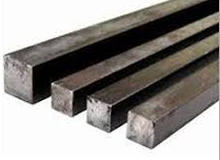### Calculating the weight of the MS square bar./ How to calculate the weight of MS square bars of all dimensions?.

Let us consider an MS square bar of size 20mm × 20mm having 3m. length.

Given data:

Width of the square bar = depth = 20mm.,
Length of the square bar = 3m.

Calculation:

The weight of the MS square bar is calculated by the formula,

= [d2L / 127.39]

Here,

d = 20mm. & L = 3m.

Weight of the bar

= [20mm × 20mm ×3m / 127.39]

= 9.42 kg.

Note: In this formula, the sectional dimension should be in millimeters & the length should be in meters.MS square bar.

So, you can calculate the weight of the MS square bars of any dimension by using this formula.

Now the question is,

#### How the formula  d2L / 127.39 is derived for the MS square bar?

Let us consider a square bar of sectional dimension d in mm having the length L as shown below.

As you know, in square bars, all 4 cross-sectional sides are equal.

The volume of the MS square bar

= [breadth × depth × length]

= [(d × 0.001) m. × ( d × 0.001) m. ×  L m.]

(1mm = 0.001m. and we have converted all dimensions into the same unit i.e. meter.)

= 0.000001 d2L cum.

Now,

the weight of the MS bar

= [volume × density]

= [0.000001 d2L cum  × 7850 kg/ cum]

= 0.00785 d2L kg

OR

By inverse,

Weight of the MS square bar

= d2L / 127.39 kg.

To go through the articles on Estimation & calculation in civil engineering, click here.